## ISIS 2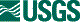ISIS Application Documentation

# interestcube

Printer Friendly View | TOC | Home

Create interest cube

 Overview Parameters Example 1 Example 2 Example 3 Example 4 Example 5

## Description

This program, interestcube, uses an interest operator to quantify how interesting each point of the input cube is. The size of the output cube matches the input cube. Each DN value of the output cube represents the quality of interest that corresponds to points in the input cube. The values of the output cube represent the quality of interest of each corresponding point in the input cube.

The user must select an interest operator (algorithm) from one of the following: 1) Förstner, 2) Gradient 3) Moravec and 4) Standard Deviation. The operator and its required values are specified in the input PVL parameter for interestcube. This input PVL is a text file that may be copied from the Examples section of this page.

Note: Forstner operator output will need to be manually stretched to see visual results, due to the wide range of values it creates.

## History

 Steven Koechle 2007-08-14 Original version Steven Koechle 2007-09-14 Renamed app from "Interest" -> "interest" Steven Koechle 2008-08-20 Renamed app from "interest" -> "interestcube" Sharmila Prasad 2010-04-14 Create a universal ground map of the input cube to be passed onto the Interest Operator to calculate the Emission Angle Ian Humphrey 2014-06-03 Closed the open cube to prevent errors from running sequential data in GUI. Fixes #1002. Makayla Shepherd 2015-02-06 Initialized the global variables and changed the catch in ISISMain in order to close the cube before the exception is thrown. Fixes #2124, 2125. Curtis Rose 2016-04-29 Fixed a documentation mistake. Extraneous information was removed from a command line example. Fixes #3910.

## Parameter Groups

Name Description
FROM Input cube
PVL Input PVL
TO Output cube

### Files: FROM

#### Description

Use this parameter to select the input cube.

 Type cube input *.cub

### Files: PVL

#### Description

Use this parameter to select the PVL file that gives the program parameters. Note: to make the program function properly the delta keywords and valid interest keyword will be changed to a value of 0.

 Type filename input

### Files: TO

#### Description

This cube will contain the results of the Interest Operator.

 Type cube output real

### Example 1

Using interestcube with Förstner operator.

#### Description

The use of Interest to ingest an ISIS cube and output a cube with interest values.

#### Command Line

interestcube from= ab102401.cub pvl= input.pvl to= out.cub
This example shows the use of interestcube to create an ISIS cube of interest values from a standard ISIS cube. This example will use a 3x3 boxcar using the Förstner Operator.

#### GUI Screenshot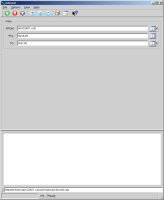Interest GUI Example GUI Screenshot of the GUI with parameters.

#### Input Image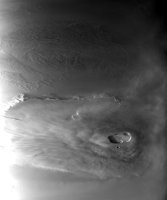This is the input ISIS cube. Input ISIS cube Parameter Name: FROM The input cube that the Interest Operator is to run on.

#### Data File

Links open in a new window.
 Input PVL file This is the PVL file that defines how the program is to operate.

#### Output Image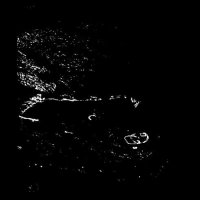Cube full of interest values after processing. Output image after processing. Parameter Name: TO The interest cube image after processing. To view a Förstner image you MUST manually stretch the image. This image has been stretched from 0 to 1.67854e-27. Keep in mind if you dont do this, the data in the cube will be valid, but will display all white in qview.

### Example 2

#### Description

The use of Interst to ingest an ISIS cube and output a cube with interest values.

#### Command Line

interestcube from= ab102401.cub pvl= input.pvl to= out.cub
This example shows the use of interestcube to create an ISIS cube of interest values from a standard ISIS cube. This example will use a 3x3 boxcar using the Gradient Operator.

#### GUI ScreenshotInterest GUI Example GUI Screenshot of the GUI with parameters.

#### Input ImageThis is the input ISIS cube. Input ISIS cube Parameter Name: FROM The input cube that the Interest Operator is to run on.

#### Data File

Links open in a new window.
 Input PVL file This is the PVL file that defines how the program is to operate.

#### Output Image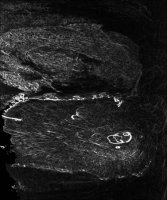Cube full of interest values after processing. Output image after processing. Parameter Name: TO The interest cube image after processing.

### Example 3

Using interestcube with Moravec operator.

#### Description

The use of Interst to ingest an ISIS cube and output a cube with interest values.

#### Command Line

interestcube from= ab102401.cub pvl= input.pvl to= out.cub
This example shows the use of interestcube to create an ISIS cube of interest values from a standard ISIS cube. This example will use a 3x3 boxcar using the Moravec Operator.

#### GUI ScreenshotInterest GUI Example GUI Screenshot of the GUI with parameters.

#### Input ImageThis is the input ISIS cube. Input ISIS cube Parameter Name: FROM The input cube that the Interest Operator is to run on.

#### Data File

Links open in a new window.
 Input PVL file This is the PVL file that defines how the program is to operate.

#### Output Image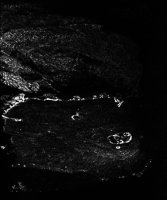Cube full of interest values after processing. Output image after processing. Parameter Name: TO The interest cube image after processing.

### Example 4

Using interestcube with Standard Deviation operator using a Small Boxcar (3x3).

#### Description

The use of Interest to ingest an ISIS cube and output a cube with interest values.

#### Command Line

interestcube from= ab102401.cub pvl= input.pvl to= out.cub
This example shows the use of interestcube to create an ISIS cube of interest values from a standard ISIS cube. This example will use a 3x3 boxcar using the Standard Deviation Operator.

#### GUI ScreenshotInterest GUI Example GUI Screenshot of the GUI with parameters.

#### Input ImageThis is the input ISIS cube. Input ISIS cube Parameter Name: FROM The input cube that the Interest Operator is to run on.

#### Data File

Links open in a new window.
 Input PVL file This is the PVL file that defines how the program is to operate.

#### Output Image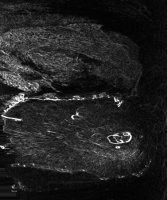Cube full of interest values after processing. Output image after processing. Parameter Name: TO The interest cube image after processing.

### Example 5

Using interestcube with Standard Deviation operator and a Large Boxcar (15x15).

#### Description

The use of Interst to ingest an ISIS cube and output a cube with interest values. This uses StandardDeviationOperator and a large boxcar to show the blurring effect of using a larger boxcar.

#### Command Line

interestcube from= ab102401.cub pvl= input.pvl to= out.cub
This example shows the use of interestcube to create an ISIS cube of interest values from a standard ISIS cube. This example will use a 15x15 boxcar using the StandardDeviationOperator.

#### GUI ScreenshotInterest GUI Example GUI Screenshot of the GUI with parameters.

#### Input ImageThis is the input ISIS cube. Input ISIS cube Parameter Name: FROM The input cube that the Interest Operator is to run on.

#### Data File

Links open in a new window.
 Input PVL file This is the PVL file that defines how the program is to operate.

#### Output Image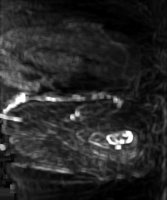Cube full of interest values after processing. Output image after processing. Parameter Name: TO The interest cube image after processing. You can see how the interest areas are slightly blured due to the large boxcar size.